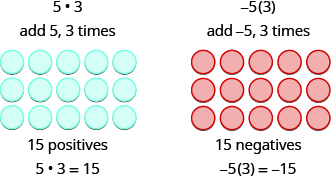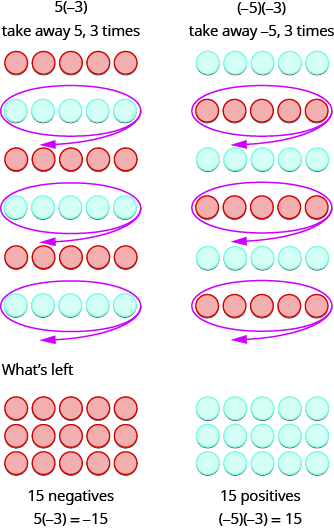Multiplying Integers

Learning Outcomes

• Multiply integers with different signs
• Multiply integers with the same sign
• Multiply an integer by -1

Since multiplication is mathematical shorthand for repeated addition, our counter model can easily be applied to show multiplication of integers. Let’s look at this concrete model to see what patterns we notice. We will use the same examples that we used for addition and subtraction.

We remember that $a\cdot b$ means add $a,b$ times. Here, we are using the model shown in the graphic below just to help us discover the pattern.Now consider what it means to multiply $5$ by $-3$. It means subtract $5,3$ times. Looking at subtraction as taking away, it means to take away $5,3$ times. But there is nothing to take away, so we start by adding neutral pairs as shown in the graphic below.In both cases, we started with $\mathbf{\text{15}}$ neutral pairs. In the case on the left, we took away $\mathbf{\text{5}},\mathbf{\text{3}}$ times and the result was $-\mathbf{\text{15}}$. To multiply $\left(-5\right)\left(-3\right)$, we took away $-\mathbf{\text{5}},\mathbf{\text{3}}$ times and the result was $\mathbf{\text{15}}$. So we found that

$\begin{array}{ccc}5\cdot 3=15\hfill & & -5\left(3\right)=-15\hfill \\ 5\left(-3\right)=-15\hfill & & \left(-5\right)\left(-3\right)=15\hfill \end{array}$

Notice that for multiplication of two signed numbers, when the signs are the same, the product is positive, and when the signs are different, the product is negative.

Multiplication of Signed Numbers

The sign of the product of two numbers depends on their signs.

Same signs Product
•Two positives•Two negatives

PositivePositive

Different signs Product
•Positive • negative•Negative • positive

NegativeNegative

example

Multiply each of the following:

1. $-9\cdot 3$
2. $-2\left(-5\right)$
3. $4\left(-8\right)$
4. $7\cdot 6$

Solution:

 1. $-9\cdot 3$ Multiply, noting that the signs are different and so the product is negative. $-27$
 2. $-2\left(-5\right)$ Multiply, noting that the signs are the same and so the product is positive. $10$
 3. $4\left(-8\right)$ Multiply, noting that the signs are different and so the product is negative. $-32$
 4. $7\cdot 6$ The signs are the same, so the product is positive. $42$

try it

Watch the following video for more examples of how to multiply integers with different signs, and the same sign.

When we multiply a number by $1$, the result is the same number. What happens when we multiply a number by $-1?$ Let’s multiply a positive number and then a negative number by $-1$ to see what we get.

$\begin{array}{ccc}\hfill -1\cdot 4\hfill & & \hfill -1\left(-3\right)\hfill \\ \hfill -4\hfill & & \hfill 3\hfill \\ \hfill -4\text{ is the opposite of }\mathbf{\text{4}}\hfill & & \hfill \mathbf{\text{3}}\text{ is the opposite of }-3\hfill \end{array}$

Each time we multiply a number by $-1$, we get its opposite.

Multiplication by $-1$

Multiplying a number by $-1$ gives its opposite.

$-1a=-a$

example

Multiply each of the following:

1. $-1\cdot 7$
2. $-1\left(-11\right)$